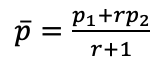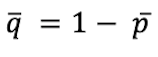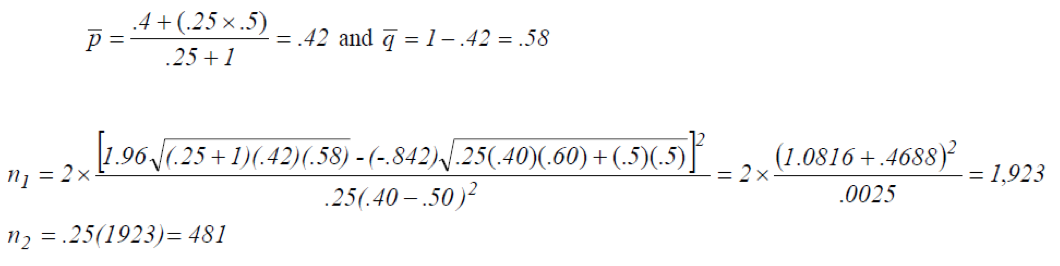Print View Documents

# Subgroup comparisons and their effect on sample size

The discussion of factors affecting sample size is based on surveys designed to obtain national and subnational estimates for the prevalence of certain nutrient-related indicators. However, sometimes an objective of the survey will be to compare subgroups, such as comparing males to females, or comparing the prevalence of a specific deficiency among a defined population group in households that do not use a fortified food to the equivalent estimate in households that do. If these types of comparison are important, then the sample size will usually need to be increased to ensure that precision for each subgroup is adequate to make a reliable comparison.

If it is expected that the two subgroups are fairly equally distributed in the population (such as females and males in most populations), then the sample size presented in the previous section could be used with substitution of the estimated proportion for the indicator for the two subgroups as the values for p1 and p2. If the two subgroups differ in size, another sample size formula should be used.

Suppose the prevalence of anaemia among WRA in households using iron-fortified flour is to be compared to the prevalence of anaemia among WRA in households not using iron-fortified flour, and it is estimated that 80% of households in the population use iron-fortified flour. The sample size formula is:

Where:andwhen sample sizes are to be unequal.

The term r is an element added to the previous formula for calculating sample size. In this example, r would be the proportion of households not using iron-fortified flour divided by the proportion of households using iron-fortified flour. In the above example, r = 0.2 ÷ 0.8 = 0.25.

Two sample sizes are calculated:
n1 = the number of households using the fortified product
n2 = the number of households not using the fortified product

For example, assume the following:
p1 = 0.40, the prevalence of anaemia in households using iron-fortified flour
p2 = 0.50, the prevalence of anaemia in households not using iron-fortified flour
r = 0.2 ÷ 0.8 = 0.25
α = 0.05, therefore Zα/2 = 1.96
β = 0.20, therefore Z1-β = -0.842
DEFF = 2Therefore, the survey would need to include 2404 individuals, of whom 1923 would be expected to be from households that use iron-fortified flour and 481 would be expected to be from households that do not use iron-fortified flour.